Morgado, T. F. M., Dias, A. M. P. G., Machado, J. S., and Negrão, J. H. (2012). "SIMPOLE -- Simulation of wood poles mechanical behaviour," BioRes. 7(3), 3439-3451.

#### Abstract

Generation of virtual poles, based on the statistical distribution of selected visual and physical properties, and the estimation of their mechanical properties are of great help for predicting the quality of the roundwood that a forest stand can provide. With this objective in mind, an algorithm, SIMPOLE (SIMulator of POLEs), was developed. This algorithm allows the generation of pole’s geometry, as well as the mechanical properties of clear wood and the distribution of knots along its length. This generation is made through statistical distributions. The generated poles are simulated, either according to standard EN14251 or according to EN14229, for testing with simple supported beam conditions or with cantilever beam conditions, respectively. The algorithm outputs are: the bending strength, the modulus of elasticity, the location, and cause of failure. The algorithm was calibrated with a sample of 56 maritime pine utility poles and validated with another sample of 57 small diameter maritime pine poles. The results for the validation sample show: for bending strength, a mean error of 16.9%, and for modulus of elasticity, a mean error of 17.2%. Based on these results, the algorithm shows potential to estimate mechanical characteristics of small diameter poles from a forest.

SIMPOLE – SIMULATION OF WOOD POLES MECHANICAL BEHAVUOUR

Telmo F. M. Morgado,a Alfredo M. P. G. Dias,a,* José S. Machado,b and João H. Negrão a

Generation of virtual poles, based on the statistical distribution of selected visual and physical properties, and the estimation of their mechanical properties are of great help for predicting the quality of the roundwood that a forest stand can provide. With this objective in mind, an algorithm, SIMPOLE (SIMulator of POLEs), was developed. This algorithm allows the generation of pole’s geometry, as well as the mechanical properties of clear wood and the distribution of knots along its length. This generation is made through statistical distributions. The generated poles are simulated, either according to standard EN14251 or according to EN14229, for testing with simple supported beam conditions or with cantilever beam conditions, respectively. The algorithm outputs are: the bending strength, the modulus of elasticity, the location, and cause of failure. The algorithm was calibrated with a sample of 56 maritime pine utility poles and validated with another sample of 57 small diameter maritime pine poles. The results for the validation sample show: for bending strength, a mean error of 16.9%, and for modulus of elasticity, a mean error of 17.2%. Based on these results, the algorithm shows potential to estimate mechanical characteristics of small diameter poles from a forest.

Keywords: Simulation model; Stochastic models; Roundwood; Poles; Mechanical properties

Contact information: a: Departamento de Engenharia Civil, Faculdade de Ciências e Tecnologia, Universidade de Coimbra, , Rua Luís Reis Santos – Pólo II da Universidade, 3030-788 Coimbra, Portugal; b: Laboratório Nacional de Engenharia Civil – LNEC, Núcleo de Estruturas de Madeira, Av. Do brasil, 101, 1700 – 066 Lisboa, Portugal.; *Corresponding author:alfgdias@dec.uc.pt

INTRODUCTION

Tools that allow the determination of modulus of elasticity and bending strength of roundwood are often used to estimate the characteristic values of the mechanical properties of logs from a forest stand. Three types of models are generally found: models that allow the estimation of mechanical properties, models that can simulate the longitudinal variability of the mechanical properties, and finally, models that can generate visual features in logs. This paper presents an algorithm that combines all three types of stated models.

A model of the lengthwise variation of bending strength in a timber beam was presented by Riberholt and Madsen (1979). It is assumed in this model that the bending strength is constant, similar to that of clear wood, except when defects occur. A similar model was later presented (Czmoch et al. 1991), assuming that a piece of timber is composed of short weak zones connected by sections of clear wood. The weak zones are composed of knots or groups of knots and are randomly distributed. The strength of the weak zones is randomly generated based on a distribution function of strength of weak zones. The bending strength variability has been simulated by Isaksson (1999), based on the previous study of Riberholt and Madsen (1979). The statistical model was then used to generate the bending strength variability of beams with the following stochastic variables i) distance between defect segments, ii) length of defect segments, iii) bending strength of defect segments, and iv) bending strength of clear wood segments.

The longitudinal variability of modulus of elasticity has been simulated by Kline et al. (1986). The authors used a second order Markov process, based on data from bending tests on the four central 76.2 mm segments of 4.88 mm timber beams, to generate serially correlated modulus of elasticity values along 76.2 mm segments of a piece of timber. Showalter et al. (1987) extended the previous model for generation of tension strength. The model generates tension strength values for each 76.2 mm segment along the length of the piece, the piece tension strength being the lowest value generated. Lam and Varuglu (1991) presented a similar model for generation of tension strength but in 610 mm segments along beams of 6.1m length of spruce-pine-fir. The model uses the standard deviation of the tension strength along the length, the correlation between the minimum and mean tension strength, and the correlation between segments tension strength to generate tension strength values along the length of the beam. The variability of compression strength has been simulated along the length of sawn spruce-pine-fir timber by Lam and Barrett (1992). The model uses the correlation structure between the compression strength values of segments within a piece of timber to estimate the compression strength of segments with unknown compression strength values.

The final type of model found is about the generation of visual features. Winn et al. (2004) developed a computer program, ALOG (Artificial Log Generator), built in Microsoft® Excel, for the generation of virtual logs for input in sawing simulation programs. This program, based on statistical distribution, allows for the random generation of length, taper, sweep, knots, and their location in the log surface.

The objective of the present work was to develop an algorithm to predict the mechanical properties of the roundwood that a forest stand can provide. The developed algorithm combines the aforementioned models, by allowing the generation of virtual poles, based on statistical distributions of poles diameter, taper, density, and knots, and the estimation of the poles mechanical properties based on the generated features. The breakthrough is essentially related with the influence of the configuration of knots in the mechanical properties. The proposed algorithm, SIMPOLE (SIMulator of POLEs), was built in Microsoft® Excel, namely in VBA (Visual Basic for Applications). It is a mathematical and probabilistic model with Monte Carlo simulations that allows the evaluation of modulus of elasticity and bending strength of poles. Two modules compose the algorithm: one module that comprises the generation of virtual poles, and another module where the virtual poles are tested.

In the generation module, the geometry, density, and knot distribution of poles are generated based on statistical distributions. Geometry of poles includes diameter, taper, and length. In terms of knots, the algorithm generates its longitudinal spacing, number, diameter, and their radial position in the transversal section. Another possibility available in the algorithm is the definition of features instead of its random generation.

In the testing module, the modulus of elasticity and bending strength are determined for the generated/inserted poles in the previous module. To that end, poles are divided in segments of clear wood and segments with knots, based on longitudinal spacing of knots and their diameter. Clear wood mechanical properties, if not inserted by the user, are obtained by correlation with the density of the pole. Mechanical properties of segments with knots are obtained from the clear wood properties using penalty factors based on the existing knots configuration. After that, poles are simulated, as an element composed by elements with different mechanical properties, for the determination of the modulus of elasticity, bending strength, and location of failure.

ALGORITHM PROPERTIES AND PARAMETERS

In this section, the description of the methodologies used in the algorithm for the generation and simulation of the poles are presented. Moreover, the decisions regarding some assumptions and simplifications, supported by bibliographic background, are also discussed.

The simulation of the poles for the determination of their mechanical properties assumes an elastic -perfectly plastic behaviour in compression and constant strength and modulus of elasticity unless defects occurs, similarly to the study by Riberholt and Madsen (1979). Hence, a wood pole was considered as an element composed of segments with defects connected by segments of clear wood.

The assignment of strength to the segments with defects could be achieved by generating the weak segments strength based on a statistical distribution, or generating the configuration of defects and based on it the weak segments strength. The first option implies the availability of data for the weak section strength distribution and, consequently, a database of weak sections strength. Such data usually are not available, so the second option was followed.

Generating defects and quantifying their penalization on strength is a complex task since roundwood has various strength-reducing defects that are difficult to model and quantify in the algorithm. Since knots are considered the major strength-reducing defect (Hoffmeyer 1987), which was confirmed in a previous study for Portuguese maritime pine timber where knots were the defect with best correlation with strength (Morgado et al. 2009), it was decided to consider only this defect in the algorithm.

The reduction in the mechanical properties in sections with knots when compared to sections of clear wood are due to various reasons, namely: i) reduction of section clear wood, ii) slope of grain around knots, iii) stress concentration, and iv) eccentric section stress distribution because of eccentric knots (USDA 1999). Furthermore, the influence of knots also depends on various reasons, namely: i) on their size, ii) on their location, and iii) stress type (the influence is higher in tension than in compression) (Newlin and Johnson 1924).

Slope of grain is considered to be the most important knot strength reduction characteristic. This occurs due to the anisotropic nature of wood, for which the strength parallel to the grain direction is significantly higher than that perpendicular to the grain direction. Goodman and Bodig in 1978 (apud Foley 2003) presented a theory for determination of slope of grain variation around knots, known as “Flow Grain Analogy.” This theory compares the fibres to the flow of a fluid around an elliptic obstacle. Franco and Pellicane (1993), using the finite element method and flow grain analogy, studied the area of influence that a knot has in the surrounding fibres which, in accordance with the study, is given by Equation , being the maximum slope of grain around a knot given by Equation .

The influence of the slope of grain on the bending strength of the wood is usually estimated using the Hankinson formula, which was originally developed for compression, but has been successfully extended for other mechanical properties (e.g. tension, bending, and modulus of elasticity) (USDA 1999; Foley 2003).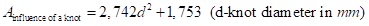(1)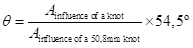(2)

Xu (2002) presented a model based on the rule of mixtures, including the proportion of volume of knots and clear wood, to estimate the effect of knots in modulus of elasticity, taking into account the angle between branches and the stem. It was considered that knots were cones with the vertex in the pole longitudinal axis. Nevertheless, this approach neglects the position of the knots in the transversal section. A way to overcome this limitation is presented by Hatayama (apud Foley 2003). The author presents a method to determine the bending strength on timber elements with knots. The elements are divided in strips; a bending strength value is assigned to each strip, with the Hankinson formula, based on the slope of grain due to the knot in that strip, estimated with an empirical formula. Then, the element is loaded, and each time the bending strength of a strip is reached, that strip is excluded and the stress redistributed, until failure of all strips.

Based on the studies presented above, a mixed approach was selected to model the effects of knots in the algorithm. Hence, knots were considered as a cone with the vertex in the pole longitudinal axis, similar to the study presented by Xu (2002). As a simplification, the cones were considered perpendicular to the pole longitudinal axis, being in the same section. Moreover, the effects of the knots on the mechanical properties were accounted for based on the referred work of Franco and Pellicane (1993) and Hatayama (apud Foley 2003). Furthermore, to take into account the different effect of a knot in the compression side and in the tension side, it was considered that knots only resist in compression. A knot in tension is considered to function like a hole. This approach will be detailed further below. Finally, the eccentricity of the section due to eccentric knots and the stress concentrations around them were not taken into account.

As mentioned earlier, the pole is considered an element with segments with knots connected by segments of clear wood. The length of segments with knots is considered to be equal to the diameter of the area of influence (dAinfluence), as determined by Equation , of the largest knot in the segment. In the transversal section, it was admitted that the dAinfluence is described along the section perimeter (Fig. 1). The length of the segments of clear wood was determined deducting to the longitudinal spacing between the centres of sections with knots half the length of these sections with knots.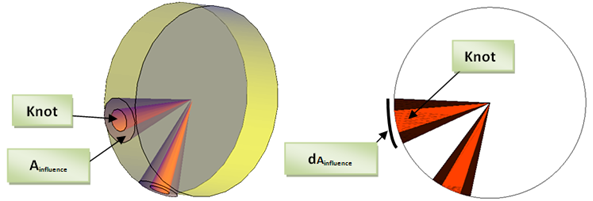Fig. 1Schematic representation of knots and their influential area

The clear wood bending strength is introduced into the model using the correlation between this property and the pole density. Since the coefficient of correlation is not unitary, the determination of bending strength should take into account this uncertainty. Hence, bending strength is generated as a random number; following a lognormal distribution (JCSS 2006) with a mean equal to the value obtain by the regression equation (bending strength and density) and standard deviation equal to the standard error of the estimate. For modulus of elasticity the procedure is similar, but it uses the correlation with bending strength instead of density, because bending strength was the variable that showed the highest coefficient of correlation with modulus of elasticity (r = 0,76) in a previous study on small-diameter maritime pine poles (Morgado et al. 2009).

The strength and modulus of elasticity of each strip is considered constant and is obtained through a weighted mean value based on the areas of knot and clear wood, and their respective bending strength and modulus of elasticity. The bending strength and modulus of elasticity of the area of influence of knots, as stated before, could be determined with Hankinson formula, Equation , which requires the following values:

• The value of the clear wood property in the grain direction, fmclear_wood.
• The ratio between that property and the one in the perpendicular direction, fm,0/fm,90. Wood Handbook (USDA 1999) recommends a value between 10 and 25 for bending strength, and a value between 8 and 25 for modulus of elasticity. From standard EN338 (CEN 2003b), we obtain a value of 30 for modulus of elasticity.
• For the exponent n, Wood Handbook (USDA 1999) recommends a value between 1.5 and 2, for bending strength, and a value of 2 for modulus of elasticity.
• The angle θ of the slope of grain around the knot, obtained from Equation (2).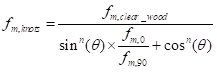Since there is a range of values for fm,0/fm,90 and for exponent n, the values of these parameters will be obtained through calibration, which will be presented in the next point of this paper. Hence, if the strip is located in the tension side, the strength of the strip is given by Equation . Alternatively, if the strip is located in the compression side, it is given by Equation . The difference results from considering, as stated earlier, that knots do not contribute to strength in tension but only in compression. A similar approach was followed for modulus of elasticity.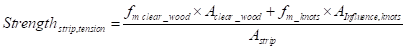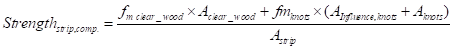As previously stated, the model comprises two modules – a generation module where the poles and their features are generated and a testing module where the mechanical tests of the generated poles are simulated for the estimation of the mechanical properties.

The generation of poles requires the generation of random numbers following a given statistical distribution. Generally, computers only generate random numbers following a uniform distribution in the range [0,1]. For generation of values following normal or lognormal distributions, it is necessary to use an auxiliary algorithm. For that purpose, the polar algorithm was selected (Ripley 1987). Using this algorithm, it is possible to generate a number following a distribution with a certain mean and standard deviation through two independent random numbers following a uniform distribution in the range [-1,1].

SIMPOLE generates the taper, density, and largest diameter of a pole, based on the random number procedure mentioned above, following a normal or lognormal distribution. After that, and with similar procedure, the algorithm generates the longitudinal spacing between sections with knots, from which pole is divided in segments of clear wood and segments with knots. In each segment with knots, the number of knots and their radial position in the section is generated, assuring no interception between knots. In Fig. 2, a summary of the generation module procedure is presented.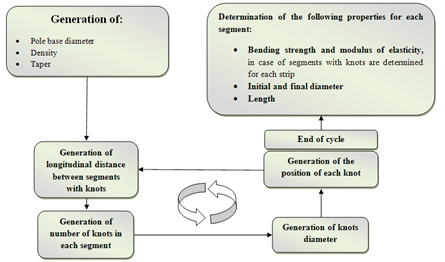Fig. 2. Schematic of the generation algorithm

The generated poles are simulated, in the testing module, according to the testing procedure proposed by the European standard EN14251 (CEN 2003a), for a simply supported beam test (Fig. 3), or EN14229 (CEN 2010) for a cantilever beam test (Fig. 4). Simulated poles are considered a one-dimensional element with three degrees of freedom, in which diameter has a uniform variation between the base and the top, according to a defined taper.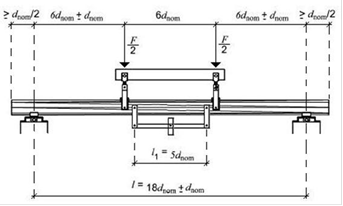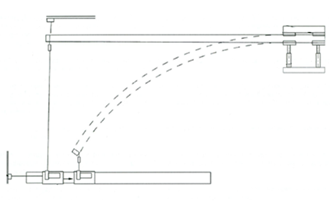Fig. 3. Simply supported beam test Fig. 4. Cantilever beam test

In case of a simply supported beam test, the determination of the displacements needed for assessment of global modulus of elasticity (Eglobal) and local modulus of elasticity (Elocal) was made with the integration of the curvature equation.

However, since the diameter of the pole is not the same along its length and the same for modulus of elasticity, the integration of the curvature equation cannot be applied directly. To overcome this problem, the pole was divided in segments of a length where it was possible to consider that in that element, the modulus of elasticity and moment of inertia are constant. Hence, the integration of the curvature equation is applied to each of these elements, being the equation of bending stress, dependent on the position of the element. However, the value of φ0 (Fig. 5) is needed for this procedure. The determination of this parameter was done with an iterative approach, with the Newton – Raphson method (Devries 1993).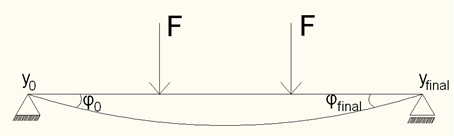Fig. 5. Schematic of the deformed shape

For determination of bending strength, the pole is subjected to load increments, defined to guarantee an error lower than 1% in value of the failure load. In each load increment, verification of stresses along the length of the pole is performed. In segments of clear wood, failure occurs when the stress exceeds the clear wood bending strength. In segments with knots, it is necessary to verify the stress in each strip of the section, since the failure only was assumed when the failure of all strips in the tension side occurs. Therefore, when the stress on a strip exceeds the strip strength, two situations must be considered: failure of the strip, if it is located in tension, or yielding of the strip, if is located in compression.

In case of a failure of a strip, the strength is set to zero; in case of yielding of a strip, the stress is kept constant, equal to that strip strength. In either case, the failure of the section would not occur if, after stress distribution, the remaining strips could support the additional stress. In order to assess that, each time a failure or yielding of a strip occurs, the algorithm calculates the new section stress diagram (Fig. 6). When the failure of a section is reached, the calculation stops and the algorithm gives as outputs: the bending strength of the pole, the location of failure, and whether it was in a segment of clear wood or with knots.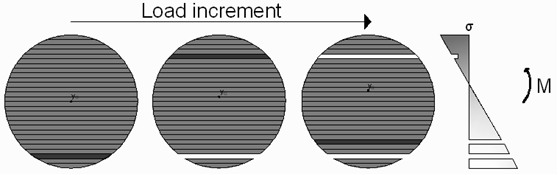Fig. 6. Section stress diagram evolution due to failure of yielding of strips (red strips) after load increments

In case of a cantilever beam test, according to EN 14229 (CEN, 2007), in the determination of the modulus, the elasticity requires the vertical and horizontal displacement on the free end of the cantilever beam. In this case, large deformations take place and theories assuming small displacements are not valid anymore. This non-linear problem was addressed by Fertis (2006), who presented a non-linear approach for these conditions. This approach was used here after some changes necessary to account for the conditions with round tapered beams.

The procedure for determination of bending strength is similar to that presented for the simply supported beam case, being the verification of bending stress made along the length of the utility pole. However, the applied bending stress is determined based on the projection of the deformed shape of the utility pole (Fig. 7).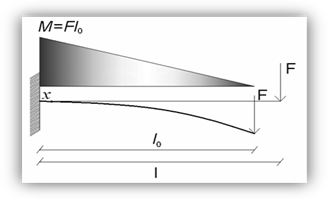Fig. 7. Bending stress on the deformed shape

To overcome this problem, Equation was used, obtained by Fertis (2006), which gives the relation between the coordinates of a point in the undeformed shape and the same point in the deformed shape, with an error lower than 3%.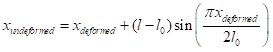MODEL CALIBRATION AND VALIDATION

Calibration

The algorithm was calibrated with a sample of 56 utility poles from a central coast region of Portugal. The wood poles were made from maritime pine roundwood (Pinus pinaster Ait). The utility poles had a diameter, at 1.5 m from the base, between 180 mm and 220 mm, a total length between 7.92 m and 8.16 m, and a mean moisture content of 65.4%. The location and size of the knots of each utility pole were recorded. This was archived by recording the longitudinal location, taking as reference the base section, and measuring the circumference from a longitudinal line that passes through the load point. After that, the utility poles were tested, as cantilever beams, for determination of bending strength (fm,0) and modulus of elasticity (E), according to EN 14229 (CEN 2010). It was observed that 76.8% of the failures were in sections with knots. Further details about these tests can be found in Martins and Dias (not published).

After the test, a slice was cut next to failure for determination of moisture content and density. Two segments of 45 cm length, one in each side of the failure, were used for determination of clear wood mechanical properties. From each segment, a specimen with 20 x 20 x 380 mm was obtained from the exterior part of the segment. The determination of the mechanical properties of the specimens followed the standard ISO 3133 (ISO, 1975).

Used as inputs for the algorithm were: i) the bending strength and modulus of elasticity of clear wood specimens, obtained as a mean of the two specimens per utility pole, ii) the geometry of the utility pole (base diameter and taper), and iii) the previous recorded location of knots. The lowest error in the estimation of mechanical properties of the calibration sample was verified with the parameters of Hankinson equation presented in Table 1. The parameter values minimize the penalization due to the knots on strength and stiffness.

A coefficient of correlation of 0.77 was obtained between the experimental bending strength of the poles and model results. A mean error of 16.6 % was associated with the prediction. The characteristic value was 20.9% inferior to the real one. In case of modulus of elasticity, the simulated value was 10.4% inferior in average to the real one. The number of failures in sections with knots was 71.4%, which was slightly inferior to experimental ones (76.8%). In 30% of the poles, the type of failure did not match the experimental results. The mechanical properties of the calibration sample and the estimations are presented in Table 2 and the failure characteristics are presented in Table 3.

Table 1. Parameters for Hankinson Formula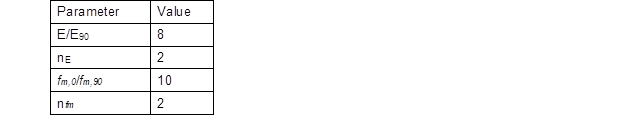Table 2. Mechanical Characteristics of Simulated Utility Poles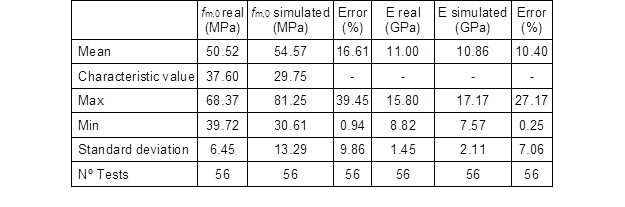Table 3. Failures Characteristics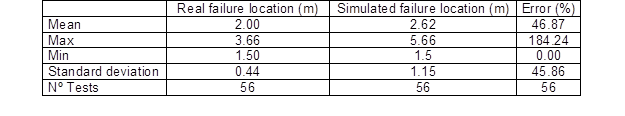Validation

In order to validate the parameters obtained through calibration, the algorithm was applied to a new sample of 57 small diameter maritime pine poles, with diameters between 100 and 150 mm and a mean moisture content of 12.1%. The poles were tested as simply supported beams, according to EN 14251 (CEN 2003a). The position of knots and clear wood mechanical properties were determined in the same way as in the calibration sample. The results of the simulation are presented in Table 4. The strength was estimated with a mean error of around 17%, similar to that obtained in the calibration set, which yields in a coefficient of correlation of 0.70 between the simulated results and the real ones. The characteristic value was 18.5% inferior to the sample. Regarding modulus of elasticity, the simulated results were 17.22%, in average, inferior to the real ones.

Since the errors in the estimation of the validation sample are similar to those obtained in the calibration, the calibration of the algorithm was judged to be successful.

Table 4. Mechanical Characteristics of Simulated Poles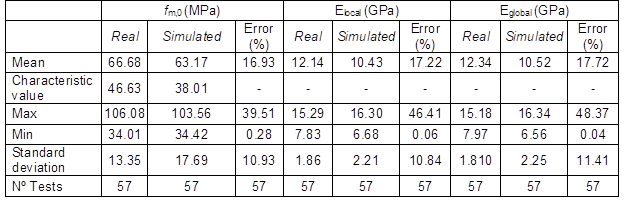CONCLUSIONS

1. The present paper presents a simulation model, SIMPOLE (SIMulator of POLEs), for predicting the quality (bending properties) of wood poles. The algorithm is composed of two modules: a generation module and a testing module. The first module comprises the generation of poles geometry, density, and distribution of knots along its length. These characteristics are either generated based on statistical distributions or can be inserted by the user. The other module allows the prediction of the ultimate load capacity, stiffness, and failure location (and if or not due to knot’s presence) considering two testing programs: according to EN14251, simple supported beam; or EN 14229, cantilever beam.
2. The algorithm was calibrated with a sample of 56 maritime pine utility poles from a Central Coast region of Portugal and tested according to EN 14229. After that, SIMPOLE was validated with another sample of 57 small-diameter maritime pine poles and tested according to EN14251. The algorithm was able to estimate the validation sample mean modulus of elasticity with an error of 14.0%. Individual modulus of elasticity of poles was estimated with a mean error of 17.7%. Bending strength of the sample was estimated with an error of 5.3% and poles bending strength with a mean error of 16.9%. The estimated characteristic value was 18.5% inferior to the real one. Based on these results, it can be concluded that SIMPOLE presents a good potential to estimate the mechanical characteristics of poles removed from a forest stand.

ACKNOWLEDGMENTS

This study has been supported by Fundação para a Ciência e Tecnologia, with the scholarship SFRH/BD/44909/2008, and by the research project PTDC/ECM/099833/ 2008.

REFERENCES CITED

CEN (2003a). “Structural round timber – Test methods,” EN14251. Comité Européen de Normalization.

CEN (2003b). “Structural timber – Strength classes,” EN338. Comité Européen de Normalization.

CEN (2010). “Structural timber – Wood poles for overhead lines,” EN 14229. Comité Européen de Normalization.

Czmoch, I., Thelandersson, S., and Larsen, H. J. (1991), “Effect of within member variability on bending strength of structural timber,” CIB W18 / 24-6-3.

Devries, P. L. (1993). A First Course in Computational Physics, John Wiley & Sons, New York.

Fertis, D. G. (2006). Nonlinear Structural EngineeringSpringer, Germany.

Foley, C. (2003), “Modeling the effects of knots in structural timber,” Division of structural Engineering, Lund Institute of Technology, Lund University, Lund, Doctoral Thesis.

Franco, N., and Pellicane, P. J. (1993), “Three-dimensional model for wood-pole strength predictions,” Journal of Structural Engineering 119(7), 2199-2214.

Hoffmeyer, P. (1987). “The role of grain angle, knots, tension wood, compression wood, and other anomalies on the mechanical properties of wood,” Technical University of Denmark, Building Materials Laboratory.

Isaksson, T. (1999), “Modelling the variability of bending strength in structural timber,” Division of structural Engineering, Lund Institute of Technology, Lund University, Lund, Doctoral Thesis.

ISO (1975). “Bois – Détermination de la résistance à la flexion statique,” ISO 3133. International Organization for Standardization.

JCSS (2006). “JCSS Probabilistic model code Part 3: Resistance models,” accessed the 9 of March, 2009, http://www.jcss.ethz.ch/publications/PMC/RESISTANCES/timber.pdf.

Kline, D. E., Woeste, F. E., and Bendtsen, B. A. (1986), “Stochastic model for modulus of elasticity of lumber,” Wood and Fiber Science 18(2), 228-238.

Lam, F., and Barrett, J. D. (1992), “Modeling lumber strength spatial variation using removal and kriging analysis,” Wood Science and Technology 26(5), 369-381.

Lam, F., and Varuglu, E. (1991), “Variation of tensile strength along the length of lumber – Part 2: Model development and verification,” Wood Science and Technology 25(5), 449-458.

Martins, C., and Dias, A. (submitted), “Bending strength of maritime pine utility poles,” Forest Products Journal.

Morgado, T., Rodrigues, J., Machado, J., Dias, A., and Cruz, H. (2009), “Bending and compression strength of Portuguese maritime pine small-diameter poles,” Forest Products Journal59(4), 23-28.

Newlin, J., and Johnson, R. (1924). “Structural timbers. Defects and their influence on strength,” Research Paper R1041, USDA Forest Service.

Riberholt, H., and Madsen, P. H. (1979). “Strength distribution of timber structures – Measured variation of the cross sectional strength of structural lumber,” Rapport R114, Technical University of Denmark.

Ripley, B. D. (1987), Stochastic Simulation, Wiley, New York.

Showalter, K. L., Woeste, F. E., and Bendtsen, B. A. (1987). “Effect of length on tensile strength in structural lumber,” Research Paper FPL-RP-482, Forest Products Laboratory.

USDA (1999), Wood Handbook: Wood as an Engineering Material, Forest Products Laboratory, Madison, pp. 4.1-4.45.

Winn, M. F., Wynne, R. H., and Araman, P. A. (2004), “ALOG: A spreadsheet-based program for generating artificial logs,” Forest Products Journal 54(1), 62-66.

Xu, P. (2002), “Estimating the influence of knots on local longitudinal stiffness in radiata pine structural timber,” Wood Science and Technology 36(6), 501-509.

Article submitted: February 23, 2012; Peer review completed: May 28, 2012; Revised version received and accepted: June 11, 2012; Published: June 19, 2012.